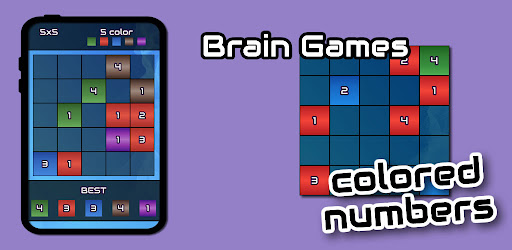Number Puzzle

## Number Puzzle Classic Colored## Mathematical puzzle

Mathematical puzzles make up an integral part of recreational mathematics. They have specific rules, but they do not usually involve competition between two or more players. Instead, to solve such a puzzle, the solver must find a solution that satisfies the given conditions. Mathematical puzzles require mathematics to solve them. Logic puzzles are a common type of mathematical puzzle.

Conway's Game of Life and fractals, as two examples, may also be considered mathematical puzzles even though the solver interacts with them only at the beginning by providing a set of initial conditions. After these conditions are set, the rules of the puzzle determine all subsequent changes and moves. Many of the puzzles are well known because they were discussed by Martin Gardner in his "Mathematical Games" column in Scientific American. Mathematical puzzles are sometimes used to motivate students in teaching elementary school math problem solving techniques. Creative thinking – or "thinking outside the box" – often helps to find the solution.

## List of mathematical puzzles

This list is not complete.

## Numbers, arithmetic, and algebra

Cross-figures or cross number puzzles
Dyson numbers
Four fours
KenKen
Water pouring puzzle
The monkey and the coconuts
Pirate loot problem
Verbal arithmetics
24 Game
Combinatorial
Cryptograms
Fifteen Puzzle
Kakuro
Rubik's Cube and other sequential movement puzzles
Str8ts a number puzzle based on sequences
Sudoku
Sujiko
Think-a-Dot
Tower of Hanoi
Bridges Game
Analytical or differential
Ant on a rubber rope
Probability
Monty Hall problem
Tiling, packing, and dissection
Bedlam cube
Conway puzzle
Mutilated chessboard problem
Packing problem
Pentominoes tiling
Slothouber–Graatsma puzzle
Soma cube
T puzzle
Tangram
Involves a board
Conway's Game of Life
Mutilated chessboard problem
Peg solitaire
Sudoku
Nine dots problem
Eight queens puzzle
Knight's Tour
No-three-in-line problem
Topology, knots, graph theory

The fields of knot theory and topology, especially their non-intuitive conclusions, are often seen as a part of recreational mathematics.

## Disentanglement puzzles

Seven Bridges of Königsberg
Water, gas, and electricity
Mechanical
Main article: Mechanical puzzle
Rubik's Cube
Think-a-Dot
Matchstick puzzle
0-player puzzles
Conway's Game of Life
Flexagon
Polyominoes